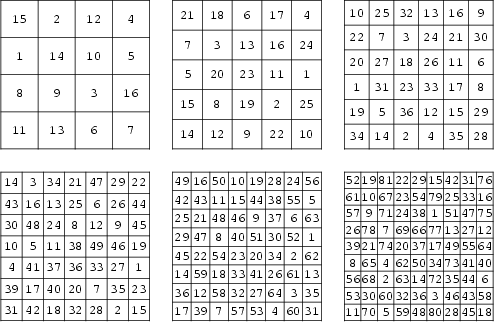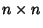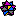## Antimagic SquareAn antimagic square is anArray of integers from 1 tosuch that each row, column, and main diagonal produces a different sum such that these sums form a Sequence of consecutive integers. It is therefore a special case of a Heterosquare.

Antimagic squares of orders one and two are impossible, and it is believed that there are also no antimagic squares of order three. There are 18 families of antimagic squares of order four. Antimagic squares of orders 4-9 are illustrated above (Madachy 1979).

References

Abe, G. Unsolved Problems on Magic Squares.'' Disc. Math. 127, 3-13, 1994.

Madachy, J. S. Magic and Antimagic Squares.'' Ch. 4 in Madachy's Mathematical Recreations. New York: Dover, pp. 103-113, 1979.Weisstein, E. W. Magic Squares.'' Mathematica notebook MagicSquares.m.